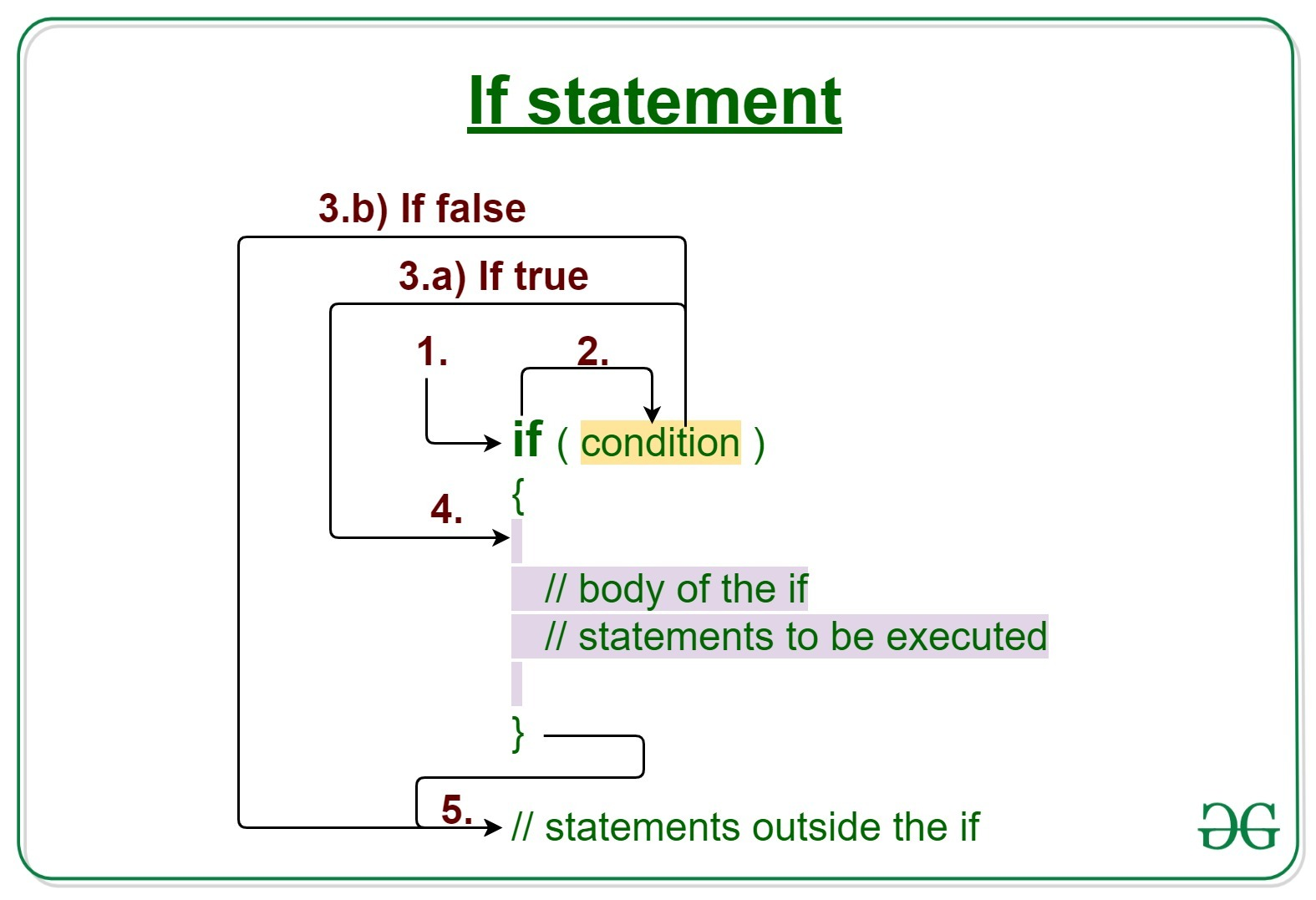Related Articles

# Java if statement with Examples

• Difficulty Level : Medium
• Last Updated : 22 Nov, 2019

Decision Making in Java helps to write decision driven statements and execute a particular set of code based on certain conditions.

The Java if statement is the most simple decision-making statement. It is used to decide whether a certain statement or block of statements will be executed or not i.e if a certain condition is true then a block of statement is executed otherwise not.Syntax:

```if(condition)
{
// Statements to execute if
// condition is true
}```

Working of if statement

1. Control falls into the if block.
2. The flow jumps to Condition.
3. Condition is tested.
1. If Condition yields true, goto Step 4.
2. If Condition yields false, goto Step 5.
4. The if-block or the body inside the if is executed.
5. Flow steps out of the if block.

Operation:
The condition after evaluation of if-statement will be either true or false. The if statement in Java accepts boolean values and if the value is true then it will execute the block of statements under it.

Note: If we do not provide the curly braces ‘{‘ and ‘}’ after if( condition ) then by default if statement will consider the immediate one statement to be inside its block. For example,

```if(condition)
statement1;
statement2;

// Here if the condition is true, if block
// will consider only statement1 to be inside
// its block.```

Example 1:

 `// Java program to illustrate If statement`` ` `class` `IfDemo {``    ``public` `static` `void` `main(String args[])``    ``{``        ``int` `i = ``10``;`` ` `        ``if` `(i < ``15``)``            ``System.out.println(``"10 is less than 15"``);`` ` `        ``// This statement will be executed``        ``// as if considers one statement by default``        ``System.out.println(``"Outside if-block"``);``    ``}``}`
Output:
```10 is less than 15
Outside if-block
```

Dry-Running Example 1:

```1. Program starts.
2. i is initialized to 10.
3. if-condition is checked. 10<15, yields true.
3.a) "10 is less than 15" gets printed.
4. "Outside if-block" is printed.
```

Example 2:

 `// Java program to illustrate If statement`` ` `class` `IfDemo {``    ``public` `static` `void` `main(String args[])``    ``{``        ``String str = ``"GeeksforGeeks"``;``        ``int` `i = ``4``;`` ` `        ``// if block``        ``if` `(i == ``4``) {``            ``i++;``            ``System.out.println(str);``        ``}`` ` `        ``// Executed by default``        ``System.out.println(``"i = "` `+ i);``    ``}``}`
Output:
```GeeksforGeeks
i = 5
```

Related Articles:

Attention reader! Don’t stop learning now. Get hold of all the important Java Foundation and Collections concepts with the Fundamentals of Java and Java Collections Course at a student-friendly price and become industry ready. To complete your preparation from learning a language to DS Algo and many more,  please refer Complete Interview Preparation Course.

My Personal Notes arrow_drop_up